# How to calculate the number of work days for a four-day workweek

Excel
When calculating the difference between two dates, you may want to exclude weekends and holidays. Excel proposes a standard formula for calculating the number of workdays for standard, five-day workweek. Also, Excel proposes a formula for calculating the number of workdays for different five-day workweek or six-day workweek.

This tip is how to calculate the number of work days for less than five-day workweek.

For example, you may need to find out how many business days should be in November 2018. This calculation should exclude Saturdays, Sundays, Mondays, and holidays: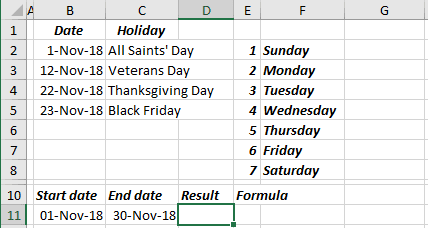1.   To count days between two dates, excluding Saturdays, Sundays and Mondays, use the formula:

= SUMPRODUCT (-- (WEEKDAY (<date range>) = <day of week>))

where:

• <date range> is an array generated from two dates, using ROW and INDIRECT formulas:

date range: ROW (INDIRECT (<start date> &":"& <end date>)),

• <day of week> taken from the WEEKDAY formula, a number between 1 and 7 that corresponds to a particular day of the week. With default settings, 1 = Sunday, 2 = Monday, 3 = Tuesday, 4 = Wednesday, 5 = Thursday, 6 = Friday and 7 = Saturday.
 = SUMPRODUCT ( -- (WEEKDAY (ROW (INDIRECT (B11 &":"& C11))) <> E2), -- (WEEKDAY (ROW (INDIRECT (B11 &":"& C11))) <> E3), -- (WEEKDAY (ROW (INDIRECT (B11 &":"& C11))) <> E8))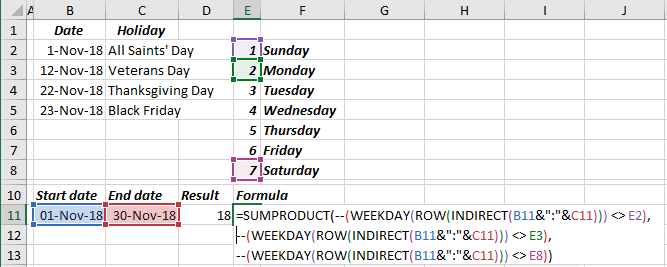• the formula (WEEKDAY (<date range>) = 1) returns the array of TRUE and FALSE,
• (-- <array>) returns array of 1 and 0 instead of TRUE and FALSE,
• SUMPRODUCT sums all values of an array.

It is easier to use ISNA and MATCH formulas instead of adding conditions three times:

= SUMPRODUCT (-- (ISNA (MATCH (WEEKDAY (<date range>), <array of week days>, 0))))

• MATCH returns the relative position of an item in an array that matches a specified value in a specified order,
• ISNA checks whether a value is #N/A, and returns TRUE or FALSE: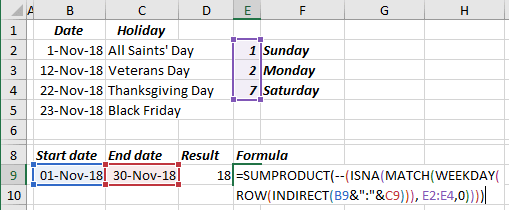So,

• the formula MATCH (WEEKDAY (<date range>), <array of weekdays>, 0) returns the array of positions for week days (Sunday, Monday, and Saturday) and the value #N/A for all other days,
• ISNA () returns an array of TRUE and FALSE for values not #N/A and #N/A,
• (-- <array>) returns array of 1 and 0 instead of TRUE and FALSE,
• SUMPRODUCT sums all values of an array.

2.   After calculating the number of working days, it is necessary to exclude all holidays:

The formula is (see How to count days of week in the range of dates):

= SUMPRODUCT (-- (WEEKDAY (<holidays>) <> <day of week>))

or

= SUMPRODUCT (-- (WEEKDAY (B2:B5) <> E2), -- (WEEKDAY (B2:B5) <> E3), -- (WEEKDAY (B2:B5) <> E4)):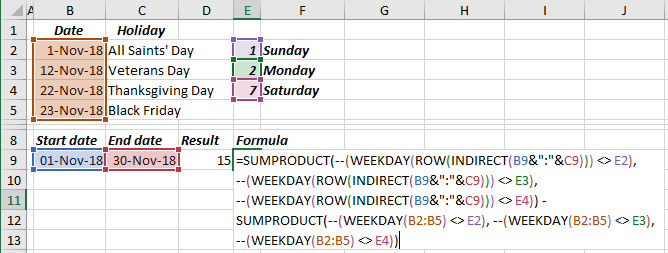With ISNA and MATCH, this formula will look shorter: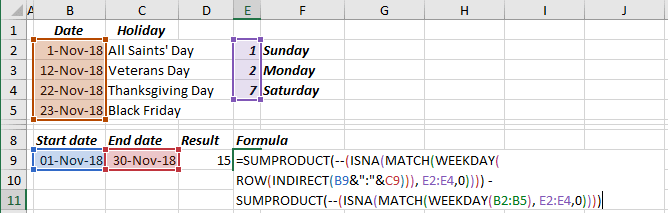See also this tip in French: Comment calculer le nombre de jours de travail pour une semaine de travail de quatre jours.